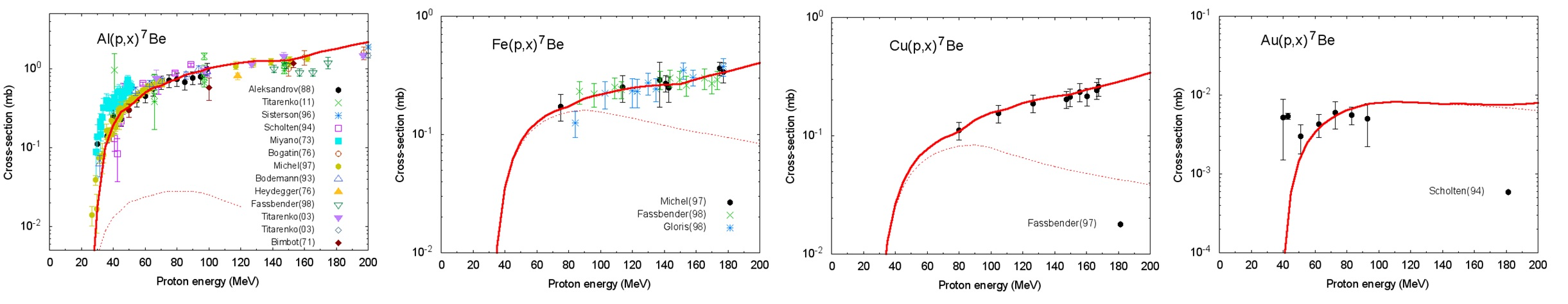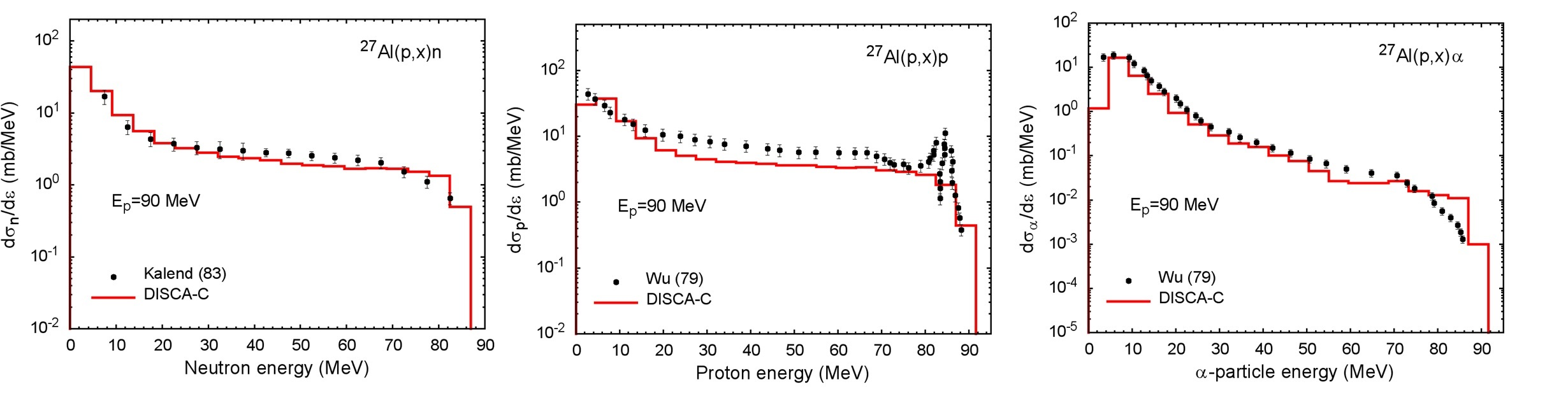# Codes for Simulation of Nuclear Reactions and Ion Irradiation of Materials

## TALYS code with GDH model

The geometry dependent hybrid model proposed by M.Blann and supplied with models for the non-equilibrium cluster emission was implemented in the TALYS code.

Go to TALYS-G page

## ALICE/ASH code with modelling of heavy cluster emission (A > 4)

Authors: M.Blann et al

The ALICE/ASH code is an advanced and modified version of the ALICE code originated by M.Blann.
The modifications concern the implementation in the code of models describing the pre-compound composite particle emission (d,t,3He,α-particle), heavy cluster emission (A > 4), fast γ-emission, different approaches for the nuclear level density calculation and the model for the fission fragment yield calculation.
The code can be applied for the calculation of excitation functions, energy and angular distribution of secondary particles in nuclear reactions induced by nucleons and nuclei with the energy up to 300 MeV.

The previous version of the code (2006) is described here. (It does not yet support cluster emission).

Many illustrations of cross-sections with the production of heavy clusters calculated using the ALICE/ASH code are given in KIT Report 7684 (2014).

Example of the calculations using current version of the code. The total cross section with the production of 7Be is shown by the solid line, and the contribution of pre-equilibrium 7Be-emission by the dashed line.Please, send a request to get the code to: alexander_konobeev ∂ kit_edu

## DISCA-C code: intranuclear cascade evaporation model with the simulation of cluster emission

The DISCA code has been elaborated to study nucleon and α-particle interactions with nuclei at intermediate energies.

The code calculates energy and angular distributions of particles emitted in nuclear reactions, residual nuclei yields, recoil spectra, total nonelastic cross-section for nuclear reactions induced by nucleons and a-particles. The code implements the advanced intranuclear cascade evaporation model with consideration of the nucleon-cluster interactions.

Example of DISCA-C calculations## IOTA code for the simulation of ion transport in materials

The code is used for the calculation of the total number of primary defects created in materials, displacement cross-sections, and spatial defect distributions. The calculations apply analytical expressions for the differential cross-section for the transfer of the kinetic energy from the moving ion to a lattice atom and tabulated stopping power data. The simulation is based on the binary collision approximation and the Monte Carlo method.

Using the results of molecular dynamics calculations or the arc-dpa model, the IOTA code is applied for joint BCA-MD or BCA-arc-dpa simulations.